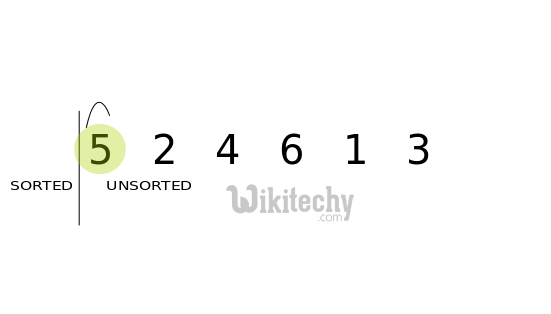# C# Swap | C# Program To Swap Two Numbers - c# - c# tutorial - c# net

## How to write Swap Program in C# ?

• When the values of two variables are exchanged at runtime it is called swapping of the two values.
•Swap numbers in csharp

• We can swap two numbers without using third variable.
• There are two common ways to swap two numbers without using third variable:
1. By + and -
2. By * and /## Program 1: Using ∗ and /

• Let's see a simple C# example to swap two numbers without using third variable.

## Program 2: Using + and -

• Let's see another example to swap two numbers using + and -.